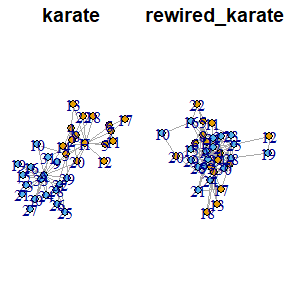# Graph Rewiring Functions

library(clustAnalytics)

## Transferred Weight Selection

In the weighted case, the switching algorithm transfers a certain amount of weight from some edges to others. The package provides two settings, which we will choose according to what type of weighted graph we are working with.

#### Complete graphs with a fixed upper bound

These graphs have an edge between every pair of vertices, which will usually be the result of applying some function to each pair. For example, networks resulting from computing correlations of time series (where each series corresponds to a vertex, and the edge weights are the correlations between series) fall into this category.

We show an example built from correlations of currency exchange time series . In this network vertices are pairs of exchange rates, and the edge weights are the correlations of their corresponding time series, scaled to the interval $$[0,1]$$. In this case, the appropriate setting is the one that keeps the variance of the edge weights constant.

data(g_forex, package="clustAnalytics")
rewireCpp(g=g_forex, weight_sel="const_var", lower_bound=0, upper_bound=1)
#> IGRAPH fcd5132 UNW- 78 5840 --
#> + attr: name (v/c), TRUE (v/c), weight (e/n)
#> + edges from fcd5132 (vertex names):
#>   SGDEUR--SGDCNY SGDAUD--SGDSEK JPYUSD--HKDMXN SGDCHF--SGDNZD JPYUSD--CNYCHF
#>  CADAUD--SGDSEK AUDEUR--SGDSEK MXNAUD--SGDSEK HKDUSD--SGDSEK AUDUSD--SGDCHF
#>  HKDUSD--SEKNZD NZDUSD--SGDNZD GBPUSD--NZDCNY AUDUSD--JPYEUR CHFUSD--AUDJPY
#>  JPYUSD--SGDNZD CNYGBP--SGDSEK CHFUSD--SGDCNY SGDUSD--SGDNZD MXNEUR--SGDNZD
#>  JPYUSD--GBPUSD MXNAUD--SGDCNY AUDJPY--SGDCHF GBPUSD--CNYGBP MXNGBP--SGDSEK
#>  GBPEUR--SGDCNY JPYUSD--SEKUSD NZDGBP--SGDCHF JPYUSD--CHFUSD CHFUSD--HKDAUD
#> + ... omitted several edges

#### More sparse graphs with weights that are non-negative but not necessarily upper bounded

This describes most commonly found weighted graphs, where the weights quantify some characteristic of the edges. Multigraphs also fit here, if we reinterpret them as weighted graphs where the edge weight is the number of parallel edges between each pair of vertices.

This is the case for the famous karate club graph from Zachary.

data(karate, package="igraphdata")
rewired_karate <- rewireCpp(karate, weight_sel="max_weight")
oldpar <- par(mfrow=c(1,2), mai=c(0,0.1,0.3,0.1)) #save original parameters
plot(karate, main="karate")
plot(rewired_karate, main="rewired_karate")par(oldpar) #restore original parameters